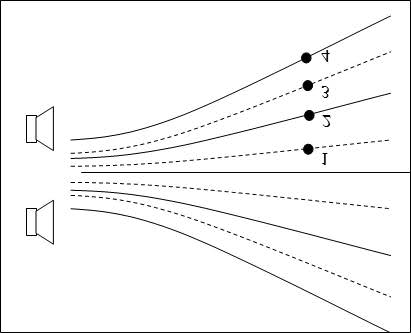# Finding wavelength of sound waves

## Homework Statement

The two speakers are producing identical sound waves. The solid lines represent constructive interference regions and the dashed lines represent destructive interference regions. The point labeled 4 is 1228.5 m from the bottom speaker and 1618.5 m from the top speaker.
What is the wavelength of the sound waves?

x-x=wavelength

## The Attempt at a Solution

1618.5m-1228.5m=390m not correct
I wasn't really sure, but this is the only thing I could think of.

## Answers and Replies

Homework Helper

What is the distance between the speaker?

this is all the information the problem gives

Homework Helper

Is there any diagram along with the problem?

The diagram is below..

Last edited:Here it is it is inverted for some reason but still...

Homework Helper

If D is the distance between the plane of the speaker and th plane of the detector, and 2d is the distance between the speakers, and x is the distance of the point 4 from the axis, then
(1228.5)2 = D^2 -x^2.....(1)
(1618.5)^2 = D^2 + x^2.......(2)
No solve for x.
From the figure, it can be written that
x = nλ and n = 2. Now find λ.

Last edited: